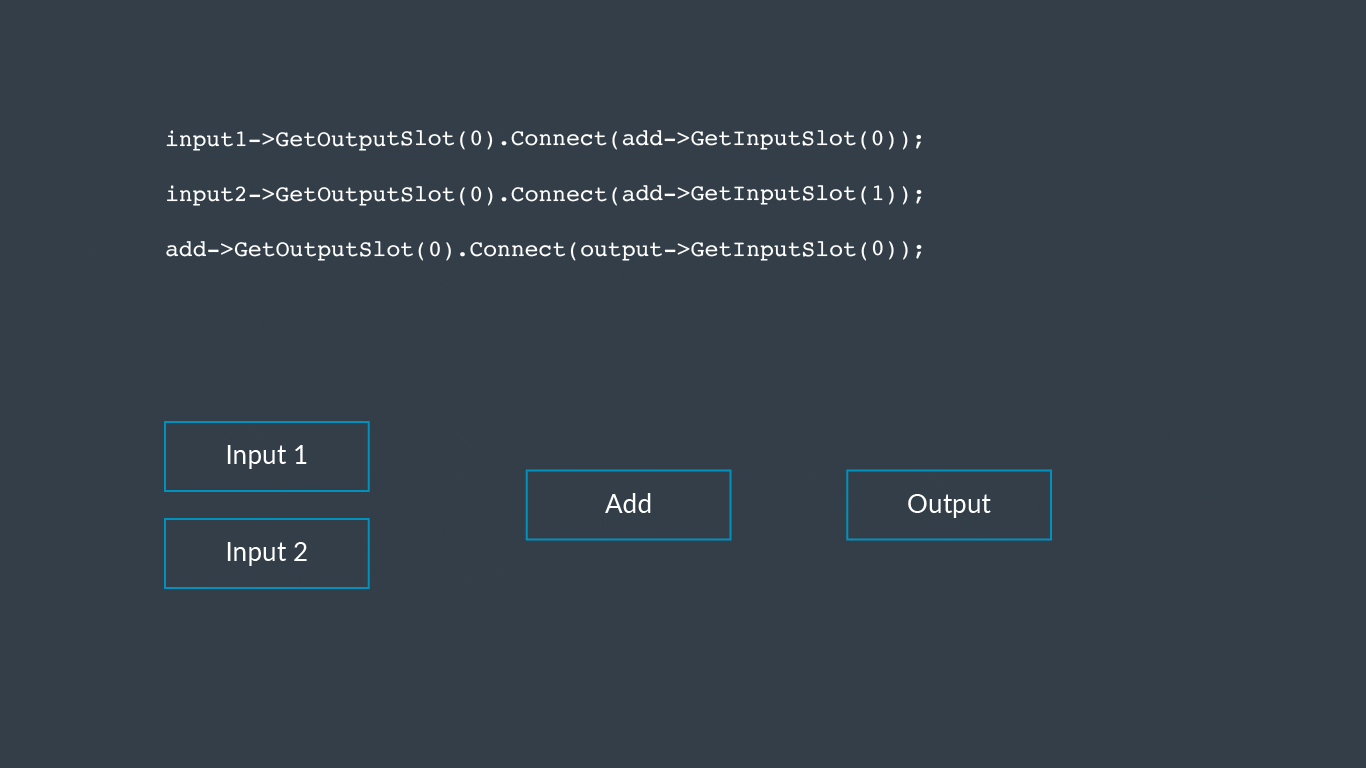## How the custom backend works

The unit tests that are included in the example custom plugin illustrate how the custom plugin works.

To see how it works, we will look at the `AdditionToPreCompiledTest()` example in the `CustomEndToEndTests.cpp` file.

The `AdditionToPreCompiledTest()` function :

• creates an initial graph with an addition layer, performing element-wise addition of vectors
• optimizes the graph. In the example, optimizing the graph substitutes the addition layer with a precompiled layer
• runs the inference on the optimized graph with some test values
• checks that the results are correct

Follow these steps to understand how the custom backend works.

1. Create an empty model object:

`INetworkPtr net(INetwork::Create());`
2. Add layers to the model:

```IConnectableLayer* input1 = net->AddInputLayer(0);
3. Create the required connections between the layers:

```input1->GetOutputSlot(0).Connect(add->GetInputSlot(0));
The following diagram shows the connections between the layers:4. Set the tensor information for each of the outputs:

```TensorInfo tensorInfo(TensorShape({3, 4}), DataType::Float32);
input1->GetOutputSlot(0).SetTensorInfo(tensorInfo);
input2->GetOutputSlot(0).SetTensorInfo(tensorInfo);
5. Optimize the completed network using the `Optimize()` function:

```IOptimizedNetworkPtr optimizedNet = Optimize(*net, defaultBackends,
runtime->GetDeviceSpec());```

The `Optimize()` function has the following specification:

`IOptimizedNetworkPtr Optimize(INetwork, {BackendId,... }, IDeviceSpec,                                    OptimizerOptions, errMessages)`

The `Optimize()` function:

• performs basic validation of the input network
• modifies the graph for correctness by:
• inserting copy layers between backends
• inserting FP32/FP16 conversion layers if necessary (specified in OptimizerOptions)
• adding debug layers, if necessary (specified in OptimizerOptions)
• performs backend-independent optimizations by:
• removing redundant operations
• optimizing all permutes and reshapes where possible
• decides which backend to assign to each layer by:
• using the `Is<x>LayerSupported()` function in the ILayerSupport interface to identify the preferred backend
• runs backend-specific optimizations by:
• for each selected backend, extracting the subgraphs that can be executed on that backend
• for each subgraph, calling `OptimizeSubGraph()` on the selected backend
6. Create and configure the runtime object:

`IRuntime::CreationOptions options;IRuntimePtr runtime(IRuntime::Create(options));`

`NetworkId networkId;runtime->LoadNetwork(networkId, std::move(optimizedNet));`
The `LoadNetwork()` function:
• creates a `LoadedNetwork` object and adds it to the runtime
• creates a list of workloads, one per layer, using the backend’s `IWorkloadFactory` object
• returns a network identifier, `networkId`, to use later for running the optimized network
8. Create sample input and output data structures:

```std::vector <float> input1Data
{
1.f, 2.f, 3.f, 4.f, 5.f, 6.f, 7.f, 8.f, 9.f, 10.f, 11.f, 12.f
};
std::vector<float> input2Data
{
100.f, 200.f, 300.f, 400.f, 500.f, 600.f, 700.f, 800.f, 900.f, 1000.f, 1100.f, 1200.f
};
std::vector<float> outputData(12);

InputTensors inputTensors
{
{ 0, ConstTensor(runtime->GetInputTensorInfo(networkId, 0), input1Data.data()) },
{ 1, ConstTensor(runtime->GetInputTensorInfo(networkId, 0), input2Data.data()) }
};
OutputTensors outputTensors
{
{ 0, Tensor(runtime->GetOutputTensorInfo(networkId, 0), outputData.data()) }
};```
9. Run the inference:

`runtime->EnqueueWorkload(networkId, inputTensors, outputTensors);`

The `networkId` is the one that is returned by the earlier call to `LoadNetwork()`.

The `EnqueueWorkload()` function executes all workloads sequentially on the assigned backends and places the result in the output tensor buffers.